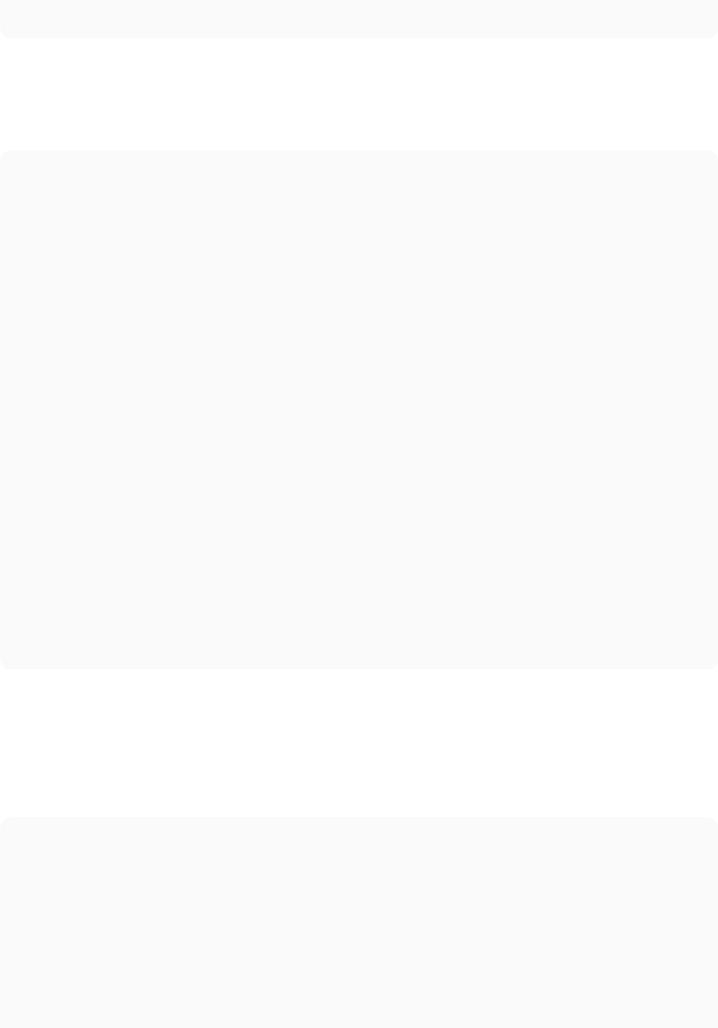11. Boolean Operators

Section 11.1: `and` and `or` are not guaranteed to return a boolean

When you use or, it will either return the ﬁrst value in the expression if it’s true, else it will blindly return the second value. I.e. or is equivalent to:

def or_(ab): if a:

return else:

return b

For and, it will return its ﬁrst value if it’s false, else it returns the last value:

def and_(ab): if not a:

return else:

return b

Section 11.2: A simple example

In Python you can compare a single element using two binary operators–one on either side:

if 3.14 3.142print(“x is near pi”)

In many (most?) programming languages, this would be evaluated in a way contrary to regular math: (3.14 x) 3.142, but in Python it is treated like 3.14 and 3.142, just like most non-programmers would expect.

Section 11.3: Short-circuit evaluation

Python minimally evaluates Boolean expressions.

>>>def true_func():

… print(“true_func()”)

… return True

>>>def false_func():

… print(“false_func()”)

… return False

>>>true_func() or false_func() true_func()

True

>>>false_func() or true_func() false_func()

true_func() True

>>>true_func() and false_func() true_func()

false_func() False

>>>false_func() and false_func()false_func() False

Section 11.4: and

Evaluates to the second argument if and only if both of the arguments are truthy. Otherwise evaluates to the ﬁrst falsey argument.

True

True

and # z = True

True

False

and # z = False

False

True

and # z = False

False

False

and # z = False

1

and # z = y, so z = 1, see `and` and `or` are not guaranteed to be a boolean

1

and # z = x, so z = 0 (see above)

0

and # z = y, so z = 0 (see above)

0

and # z = x, so z = 0 (see above)

The 1‘s in the above example can be changed to any truthy value, and the 0‘s can be changed to any falsey value.

Section 11.5: or

Evaluates to the ﬁrst truthy argument if either one of the arguments is truthy. If both arguments are falsey, evaluates to the second argument.

True

True

or # z = True

True

False

or # z = True

False

True

or # z = TrueFalse

False

or # z = False

1

or # z = x, so z = 1, see `and` and `or` are not guaranteed to be a boolean

0

or # z = x, so z = 1 (see above)

1

or # z = y, so z = 1 (see above)

0

or # z = y, so z = 0 (see above)

The 1‘s in the above example can be changed to any truthy value, and the 0‘s can be changed to any falsey value.

Section 11.6: not

It returns the opposite of the following statement:

 x = True y = not x # y = False x = False y = not x # y = True

*This content is compiled from Stack Overﬂow Documentation, and the content is written by the beautiful people at Stack Overﬂow.  This work is licensed under cc by-sa.

*This content is compiled from Stack Overﬂow Documentation, and the content is written by the beautiful people at Stack Overﬂow.